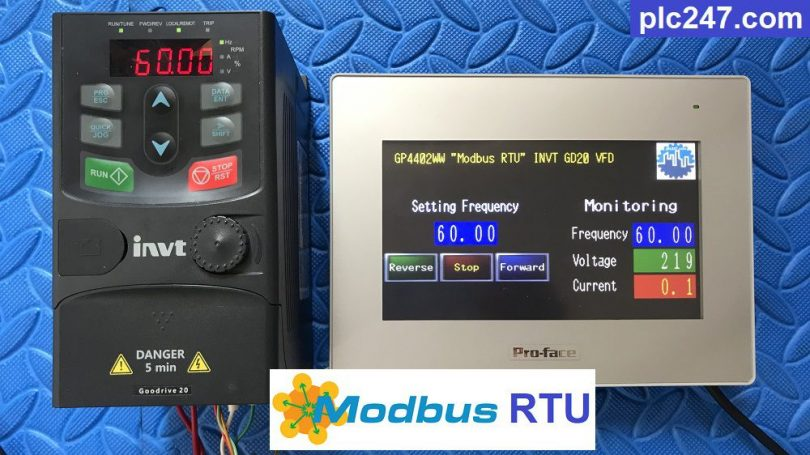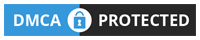# HMI Proface “Modbus RTU” INVT GD20 VFDWritten by

Hello everyone!
The pair of inverters INVT GD20 and HMI Proface GP4402WW are two devices that are used a lot in today’s machines. The low price and good quality make it the choice of many engineers.

Today plc247.com will write a tutorial for you to connect them together via Modbus RTU communication standard, both of these devices are built-in RS485.

#### INVT GD20 VFD Modbus RTU Configuration

To be able to control the inverter with Modbus RTU, you need to configure the same parameters (you can change some parameters but plc247.com recommends setting like this)

+ P00.01: 2 (Communication running command)

+ P00.06: 8 (The frequency is set by MODBUS)

+ P00.09: 0 (Select A frequency)

+ P14.00: 1 (Slave Number.1)

+ P14.01: 3 (9600bps)

+ P14.02: 1 (Digital bit checkout E,8,1 RTU)

+ P14.03: 5 (Communication answer delay)

+ P14.04: 2.0 (Communication overtime fault time)

>>> Control Address = 400001(dec) + 2000(hex)
= 400001(dec) + 8192(dec) = 408193

• Set Word 48193 = 1 >>> Forward Run
= 2 >>> Reverse Run
= 5 >>> Stop

>>> Frequency Set Address = 400001(dec) + 2001(hex)
= 400001(dec) + 8193(dec) = 408194

>>> Output Frequency Address = 400001(dec) + 3000(hex)
= 400001(dec) + 12288(dec) = 412289

>>> Output Voltage Address = 400001(dec) + 3003(hex)
= 400001(dec) + 12291(dec) = 412292

>>> Output Current Address = 400001(dec) + 3004(hex)
= 400001(dec) + 12292(dec) = 412293

#### GP-ProEX Software Communication Setting

Set the same communication parameters as INVT GD20 VFD

### Video Tutorial

======

Related Software and Documents